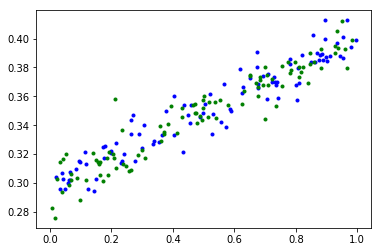This notebook demonstrates linear regression in TensorFlow, and visualizes the graph and summary data for the variables in TensorBoard. Uncomment code where instructed to learn more as you go along.

# The next three imports help with compatability between
# Python 2 and 3
from __future__ import absolute_import
from __future__ import division
from __future__ import print_function

import os

import numpy as np
import pylab
import tensorflow as tf

# A special command for IPython Notebooks that
# intructs Matplotlib to display plots in the notebook
%matplotlib inline

# This is a directory we'll use to store information
# about the graph to later visualize in TensorBoard.
# By default, it will be created in the same directory
# as this notebook.

# Be sure to delete the contents of this directory before
# running the script.
LOGDIR = './graphs'

tf.reset_default_graph()
sess = tf.Session()


This function will create a noisy dataset that's roughly linear, according to the equation y = mx + b + noise

def make_noisy_data(m=0.1, b=0.3, n=100):
x = np.random.rand(n).astype(np.float32)
noise = np.random.normal(scale=0.01, size=len(x))
y = m * x + b + noise
return x, y


Step 1) Create training and testing data.

x_train, y_train = make_noisy_data()
x_test, y_test = make_noisy_data()


Plot our training and testing data

# Uncomment the following lines to plot the data.
pylab.plot(x_train, y_train, 'b.')
pylab.plot(x_test, y_test, 'g.')

[<matplotlib.lines.Line2D at 0x7fbefbb81908>]Step 2) Define placeholders for data we'll later input to the graph.

# tf.name_scope is used to make a graph legible in the TensorBoard graph explorer
# shape=[None] means x_placeholder is a one dimensional array of any length.
# name='x' gives TensorBoard a display name for this node.
with tf.name_scope('input'):
x_placeholder = tf.placeholder(shape=[None], dtype=tf.float32, name='x-input')
y_placeholder = tf.placeholder(shape=[None], dtype=tf.float32, name='y-input')

# Uncomment the following lines to see what x_placeholder and y_placeholder are.
print(x_placeholder)
print(y_placeholder)

Tensor("input/x-input:0", shape=(?,), dtype=float32)
Tensor("input/y-input:0", shape=(?,), dtype=float32)


Step 3) Define our model.

Here, we'll use a linear model: y = mx + b

with tf.name_scope('model'):
m = tf.Variable(tf.random_normal(), name='m')
b = tf.Variable(tf.random_normal(), name='b')
# This is the same as y = tf.add(tf.mul(m, x_placeholder), b), but looks nicer
y = m * x_placeholder + b

# Uncomment the following lines to see what m, b, and y are
print(m)
print(b)
print(y)

<tf.Variable 'model/m:0' shape=(1,) dtype=float32_ref>
<tf.Variable 'model/b:0' shape=(1,) dtype=float32_ref>


Step 4) Define a loss function (here, squared error) and an optimizer (here, gradient descent).

LEARNING_RATE = 0.5

with tf.name_scope('training'):
with tf.name_scope('loss'):
loss = tf.reduce_mean(tf.square(y - y_placeholder))
with tf.name_scope('optimizer'):
train = optimizer.minimize(loss)

# Uncomment the following 3 lines to see what 'loss', 'optimizer' and 'train' are.
# print("loss:", loss)
# print("optimizer:", optimizer)
# print("train_step:", train)


Step 5) Set up TensorBoard

# Write the graph
writer = tf.summary.FileWriter(LOGDIR)

# Attach summaries to Tensors (for TensorBoard visualization)
tf.summary.histogram('m', m)
tf.summary.histogram('b', b)
tf.summary.scalar('loss', loss)

# This op will calculate our summary data when run
summary_op = tf.summary.merge_all()


Step 6) Initialize variables

At this point, our graph is complete. We're now ready to initialize variables, then begin training.

sess.run(tf.global_variables_initializer())


Step 7) Training

TRAIN_STEPS = 201

for step in range(TRAIN_STEPS):

# Session will run two ops:
# - summary_op prepares summary data we'll write to disk in a moment
# - train will use the optimizer to adjust our variables to reduce loss
summary_result, _ = sess.run([summary_op, train],
feed_dict={x_placeholder: x_train,
y_placeholder: y_train})
# write the summary data to disk

# Uncomment the following two lines to watch training happen real time.
if step % 20 == 0:
print(step, sess.run([m, b]))

# close the writer when we're finished using it
writer.close()


Step 8) See the trained values for m and b

print ("m: %f, b: %f" % (sess.run(m), sess.run(b)))


Step 9) Use the trained model to make a prediction

# Use the trained model to make a prediction!
# Remember that x_placeholder must be a vector, hence  not just 2.
# We expect the result to be (about): 2 * 0.1 + 0.3 + noise ~= 0.5
sess.run(y, feed_dict={x_placeholder: })


Step 10) Start TensorBoard

# To start TensorBoard, run this command from a terminal
# Note: you should run this from the same directory that contains this notebook
# or, provide absolute path to the 'graphs' directory
# If you are runnining in a virtualenv, be sure to start it
# if you open a new terminal window.

# \$ tensorboard --logdir=graphs

# To open TensorBoard, point your browser to http://localhost:6006
# Then, click on the tabs for 'scalars', 'distributions', 'histograms', and 'graphs'